A CYBOT TECHNICAL SITE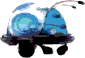# Cybot Motor Driver Board

## A description by Nigel Goodwin.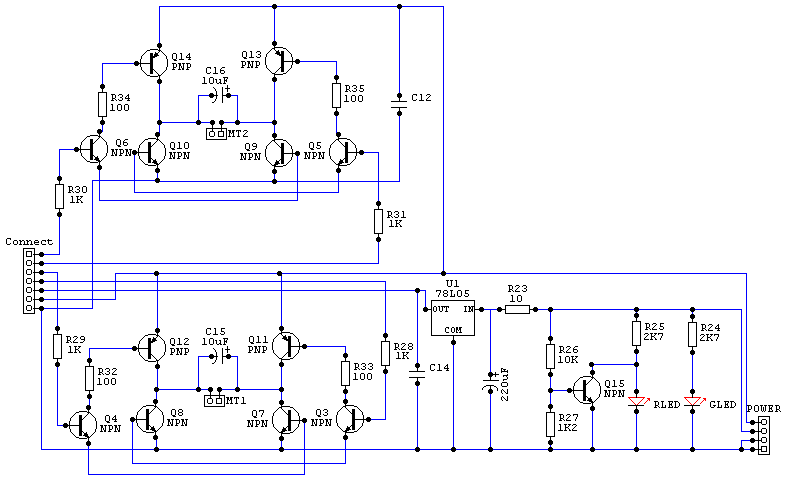Q3 - S8050 Q7 - 2SC3279 Q11 - 2SA1300 Q15 - NPN SMT Q4 - S8050 Q8 - 2SC3279 Q12 - 2SA1300 RLED - Red LED Q5 - S8050 Q9 - 2SC3279 Q13 - 2SA1300 GLED - Green LED Q6 - S8050 Q10 - 2SC3279 Q14 - 2SA1300This is the complete circuit for the Cybot driver PCB, it consists of two simple H-Bridge drivers, one for each motor, plus a 5 volt regulator for feeding power from the 9 volt battery to the control circuitry, and finally two LED's - the green one shows power is turned on (from the 9 volt battery), and the red one lights when the 9 volt battery is getting low.

 7 Pin Socket 4 Pin Socket MT1 MT2 1 - RH motor forwards. 2 - RH motor backwards. 3 - LH motor forwards. 4 - LH motor backwards. 5 - 5 volts to uP 6 - 6 volts 7 - ground. 1 - ground. 2 - ground. 3 - 9 volts in. 4 - 6 volts in. LH Motor RH MotorTo move Cybot forwards, simply take pins 1 and 3 high (which is what the motor test PCB does), to move backwards take pins 2 and 4 high, UNDER NO CIRCUMSTANCES must you take pins (1 and 2) or (3 and 4) high at the same time, this WILL destroy the output transistors!!!. To turn Cybot you have various options, to turn left you can either take pin 1 high, or take pin 4 high, or do both - doing both will allow Cybot to spin around about it's axis - a point midway between the rear wheels. To turn right you do the same, but use pins 3 and 2.

How it works?.It's a fairly straightforward circuit, the transistors are all used as simple switches. With an NPN transistor, taking the base 'positive' of the emitter will cause the transistor to switch on (diagram 2), taking the base to the same potential as (or slightly below) the emitter will cause the transistor to switch off (diagram 1) - a PNP transistor is an 'opposite' version, taking the base 'negative' of the emitter will cause the transistor to switch on. In practice a silicon transistor requires around 0.7 volts to switch on, the low battery circuit makes use of this in order to turn the transistor off when the battery is running low, but the rest of the transistors are driven 'hard on' in order to ensure they switch positively.

 Diagram 1 - NPN Off Diagram 2 - NPN On Diagram 3 - PNP Off Diagram 4 - PNP On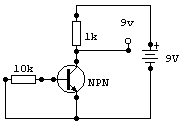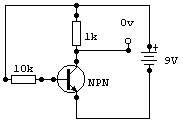So, looking at one section of the full driver circuit, Q4 and Q8 are NPN (like diagram 1 & 2), and Q12 is PNP (like diagram 3 & 4). I've redrawn this part of the circuit to make it more clear, in diagram 5 the motor is turned off, and in diagram 6 the motor is turned on. Diagram 7 shows the effect Q4 has when it's turned on, effectively connecting the 100 ohm resistor directly between the base of the two transistors.

 Diagram 5 - motor off. Diagram 6 - motor on. Diagram 7 - simplified motor on.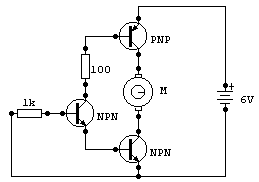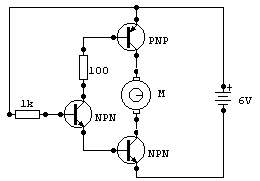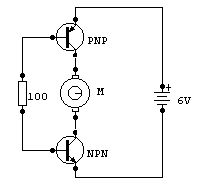Examining diagram 7 in more detail, the 100 ohm resistor connects the base of the bottom (NPN) transistor towards the positive rail, thus turning it on, at the same time it connects the base of the upper (PNP) transistor towards the negative rail, thus turning that one on as well. In this way both transistors are turned hard on, and the motor has power and rotates. With Q4 turned off, the 100 ohm in diagram 7 effectively disappears, so neither transistor is turned on, and the motor doesn't rotate. Each motor requires two sets of this circuit, one to rotate clockwise, and the other to rotate anti-clockwise, by selecting one or other of the two inputs the motor is connected either way around.

Lets go a little deeper!.I mentioned at the beginning of this article that the transistors are all turned 'hard on', this is important as it keeps the heat generated in the transistors to a low value, which makes it more efficient - and allows you to use smaller, cheaper transistors. I'll demonstrate how this works with a little bit of simple mathematics - the most important formula in Electronics is Ohms Law, which states that Voltage equals Current multiplied by Resistance - usually written V = I x R.We'll apply this formula to diagram 7, with the 100 ohm resistor - so we already know the value of 'R' = 100 ohms. So now we need to find one of the other two values - the only one we can do this with is Voltage, once we have these two we can calculate the Current using Ohms Law. Now we know the battery voltage is 6 volts, but the 100 ohm doesn't connect to the battery - BUT! it does connect  to the bases of the two transistors, whose emitters do connect to the supply rails. Now a silicon transistor drops 0.7 volts across it's Base Emitter junction, there's one at the top, and one at the bottom, both dropping 0.7 volts making a total of 1.4 volts dropped. Subtract this from the 6 volt supply and we have a total of 4.6 volts across the resistor.So now we have two parts of the formula, and we can transpose the formula to give I = V / R in order to find the current, so the current equals 4.6v divided by 100 ohms which gives a current of  0.046 amps (or 46mA). This current flows through the bases of both transistors - and a transistor works by current gain, which means the current passing through the collector is equal to the base current multiplied by the current gain of the transistor. So if we assume a current gain for the transistors as low as 100 times (it's actually considerably more than this!) we get 100 x 0.046A = 4.6A. This is more than twice the permitted current for the devices, but will be limited by the current the motor actually consumes, so the transistors are turned 'hard on' as required, for best efficiency.

The LED's.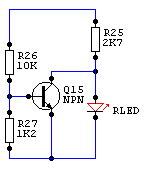Now I'll explain the LED's, as you can see from the circuit above, they are wired identically across the 9v supply, via 2.7K feed resistors. The difference is that the red LED has an NPN transistor (Q15) connected across it, this transistor is normally turned on, bypassing the current that would light the LED, thus extinguishing it. The two resistors feeding the base of Q15 (R26-10K and R27-1.2K) form a potential divider across the 9v supply so the voltage at their junction will vary with the supply voltage (or would, if the transistor wasn't there!). To work out the voltage at the junction, the formula is V = R27 x Battery/(R26+R27), which gives about 0.96v with a 9v supply. This circuit works by using the turn on voltage of a silicon transistor, which is about 0.7v, so if the base is over 0.7 volts the transistor will be on, if it's under 0.7v it will be off. To calculate this point you can transpose the formula, to give Battery = 0.7/R27 x (R26+R27) which works out at about 6.5v, so around 6.5v the low battery light will start to come on, it won't be an instant transition, but will slowly get brighter as the transistor reaches the unlinear part of it's characteristics.I hope you find this explanation helpful, if you have any queries feel free to contact me at nigelg@lpilsley.co.uk or post a question on the CybotBuilder BBS.##### Cybot graphics used by kind permission of EaglemossLast Updated 18/02/02 You can reach me by email at: nigelg@lpilsley.co.uk